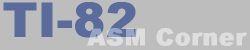Lesson 13
 TI-82 ASM Corner :: Programming Guide :: Lesson 13 Back | Home | Search
Lesson 13 : More new instructions

In this lesson, you will learn some new instructions that will be useful when you need to calculate things in your program.

These instructions add something to the A register or to the HL register. The difference between ADD and ADC is that ADC also adds the contents of the carry flag to the answer, this means that if the carry flag(CY) is set, the answer is increased by one.

Valid examples:

 ``` ADD A,A ; A = A + A ADD A,E ; A = A + E ADD A,(HL) ; A = A + (HL) ADC A,(HL) ; A = A + (HL) + CY ADD A,(IX+5) ; A = A + (IX+5) ADD A,(IY+1) ; A = A + (IY+1) ADD A,2 ; A = A + 2 ADD HL,DE ; HL = HL + DE ADC HL,BC ; HL = HL + BC + CY ```

Please note that the ADD HL,xx instructions do NOT affect the Z-flag.
The ADC HL,xx instructions affect the Z flag.

Well, suppose you wanted to add two 32 bit words and the interrupts are disabled (see lesson 20). Then you will add the least significant 16-bit words first and then you will add the most significant 16-bit words, and here you will also add the carry flag!

 ``` add hl,de exx ; hl<->hl'; de<->de' adc hl,de ```

In the previous example, the 32-bit addition of H'L'HL and D'E'DE is performed. The EXX instruction will be explained in lesson 17 and actually swaps the contents of every register with the contents of its shadow register. Please remember NOT to use EXX when interrupts are enabled!

SBC

The SBC instruction is very similar to the SUB instruction. The only difference here is that the contents of the carry flag is subtracted also. Because there is no SUB HL,xx instruction available, SBC is the only way to subtract two 16-bit registers.

Examples:

 ``` SBC A,D ; A = A - D - CY SBC A,(HL) ; A = A - (HL) - CY SBC A,(IX+1) ; A = A - (IX+1) - CY SBC A,(IY+5) ; A = A - (IY+5) - CY SBC A,3 ; A = A - 3 - CY SBC HL,DE ; HL = HL - DE - CY SBC HL,SP ; HL = HL - SP - CY ```

All these instructions affect all flags.

AND, OR and XOR

These logical operators perform the AND, OR or XOR operation on the A register. The result is stored in A.

How do this operations work?
Well, each bit of byte 1 is compared with the according bit of the other byte. The bit that will be written to the result byte will depend on those 2 bits.

See below for the characteristics of each operation.

 ``` AND --------- 1 1 -> 1 The resulting bit is 1 0 -> 0 set ONLY if both bits 0 1 -> 0 are set 0 0 -> 0 OR --------- 1 1 -> 1 The resulting bit is 1 0 -> 1 set when at least one 0 1 -> 1 of the bits is set 0 0 -> 0 XOR --------- 1 1 -> 0 Works in the same way 1 0 -> 1 as OR, but when both 0 1 -> 1 bits are set, the 0 0 -> 0 resulting bit is reset ```

Examples:

 ``` AND %10010001 OR B XOR (HL) AND (IX+5) OR (IY+7) XOR A ; LD A,0 OR A ; CP 0 ```

The last 2 examples are often used to replace LD A,0 and CP 0 because they only take ONE byte instead of two and because they are faster.

XOR A : will give A=0 as result. There's only one occasion when you shouldn't use it and that's when you need to save the state of the flags. (XOR affects the flags, LD doesn't)

OR A : A won't get destroyed and the flags will be set according to the result, which is A. So, if A was zero, the zero flag will be set.

New instructions

Here is a little table with all the new instructions from this lesson.

New instructions
Mnemonic Operation
ADD A,s A = A + s
s can be r, n, (HL), (IX+n), (IY+n)
all flags affected
ADC A,s A = A + s + CY
s can be r, n, (HL), (IX+n), (IY+n)
all flags affected
ADD HL,ss HL = HL + ss
ss can be BC, DE, HL or SP
only C flag affected
ADC HL,ss HL = HL + ss + CY
ss can be BC, DE, HL or SP
all flags affected
SBC A,s A = A - s - CY
s can be r, n, (HL), (IX+n), (IY+n)
all flags affected
SBC HL,ss HL = HL - ss - CY
ss can be BC, DE, HL or SP
all flags affected
AND s A = A && s
s can be r, n, (HL), (IX+n), (IY+n)
zero and sign flag affected, carry reset
OR s A = A || s
s can be r, n, (HL), (IX+n), (IY+n)
zero, sign and parity flag affected, carry flag reset
XOR s A = A xor s
s can be r, n, (HL), (IX+n), (IY+n)
zero,sign and parity flag affected, carry flag reset
r can be B, C, D, E, H, L, A
n is an 8-bit value in range 0-255

Previous lesson | Contents | Next lesson